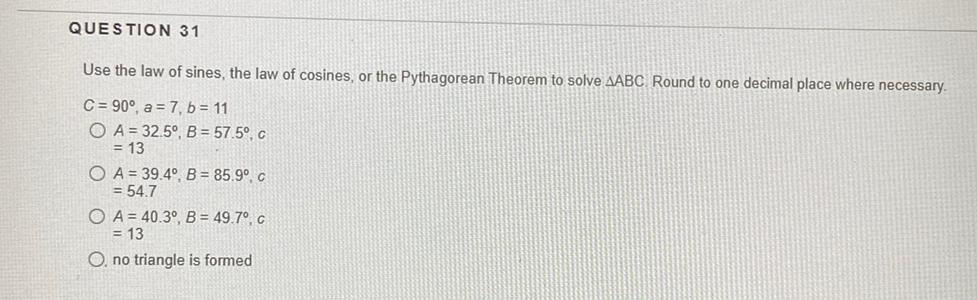Question:

# Use the law of sines, the law of cosines, or the Pythagorean Theorem to solve ∆ABC Round to one decimal place where necessary. (A) C = 90°, a = 7, b = 11 (B) A = 32.5°, B = 57.5°, c = 13 (C) A = 39.4°Use the law of sines, the law of cosines, or the Pythagorean Theorem to solve ∆ABC Round to one decimal place where necessary. (A) C = 90°, a = 7, b = 11 (B) A = 32.5°, B = 57.5°, c = 13 (C) A = 39.4°, B = 85.9°C = 54.7 (D) A = 40.3°, B = 49.7°C, c = 13 (E) no triangle is formed.